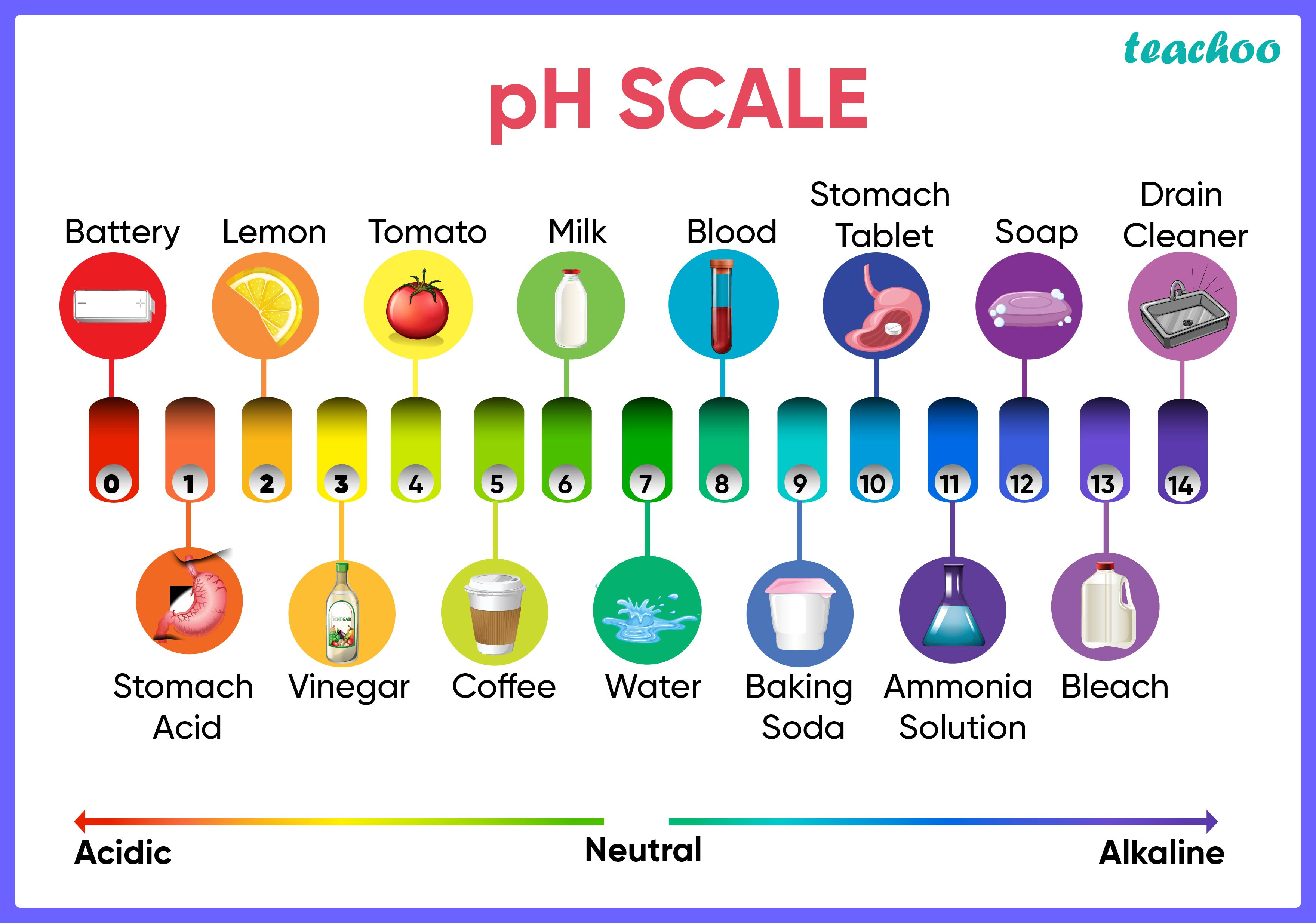CBSE Class 10 Sample Paper Science Solution - For 2023 Boards

Class 10
Solutions to CBSE Sample Paper - Science Class 10

## Anita added a drop each of diluted acetic acid and diluted hydrochloric acid on pH paper and compared the colors. Which of the following is the correct conclusion?

1. pH of acetic acid is more than that of hydrochloric acid.
2. pH of acetic acid is less than that of hydrochloric acid.
3. Acetic acid dissociates completely in aqueous solution.
4. Acetic acid is a strong acid

• pH paper is a universal indicator that is used to determine the strength of acids or bases as a function of pH (power of hydrogen) .
• The strength of an acid/base is a measure of the amount of H+ ions the solution dissociates into when added to water.
• Acids give out more H+ ions as compared to bases.
• Similarly, stronger acids dissociate into a higher amount of H+ ions than weaker acids.
• Moreover, s trong acids have lower pH values than weak acids.• We know that acetic acid is a weaker acid than HCl

Therefore, the correct answer is (a) pH of acetic acid is more than that of hydrochloric acid.

Learn in your speed, with individual attention - Teachoo Maths 1-on-1 Class